# Music Notation in 31 Tone Equal Temperament:

One of the most desirable features of 31-ET is that it is straightforward to notate using standard western musical notation. Furthermore, the notation is such that diatonic music can be directly played in 31-ET, as it was written, and it will generally sound good, with some possibly adjustments needed in the case of music that relies on enharmonic equivalents.

An added benefit of the notational convenience of 31-ET is that the standard conventions for notating Arab Music using Western musical notation (usually using semi-flats to notate intervals typically approximated with quarter tones) can be used as-is and will result in the closest-match notes and intervals from 31-ET.

## Note Names in 31-ET

31-ET consists of 31 equal divisions of the octave; all intervals can be described in terms of their number of steps on this scale. The smallest step, which serves as the building block in 31-ET, is called the diesis; when we use the term step or count the number of steps, we are counting the number of steps of this smallest interval. A basic starting point for understanding the notation of 31-ET is to understand the intervals. You may find the list of intervals in 31-ET to be a useful reference.

31-ET can be viewed as a system that starts with the familiar 12-note chromatic scale, and then breaks each note into either two or three distinct notes. The natural notes (white keys on a piano) are divided into three notes each, and the flats and sharps (black keys on a piano) are divided into two each. One helpful way to get a grasp on how notation works in 31-ET is to visualize it using a familiar piano keyboard. Imagine dividing each white key into three notes, and each black key into two notes. The new notes on the white keys are given the name semisharps and semiflats. The arrow shows the motion from one step to the next.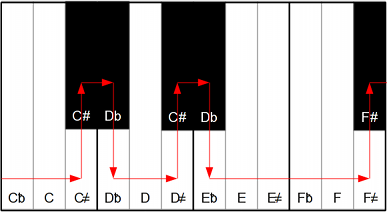## Sharps, Flats, Semisharps, Semiflats

In 31-ET, a sharp or a flat moves up two steps of the scale. A semisharp or semiflat moves up one step. As expected, a double sharp or double flat moves up or down four steps. One can also notate a sharp-and-a-half, called a sesqui-sharp, and a flat-and-a half (sesqui-flat), to move up and down three steps. The double sharp and double flat each represent a move of four steps, which, in 31-ET is one step less than a whole tone.

 Accidental: Notation: Steps: Interval: Natural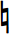0 Unison Semisharp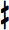+1 Diesis Sharp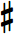+2 Chromatic Semitone Sesquisharp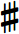+3 Diatonic Semitone Double Sharp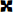+4 Neutral Second Semiflat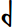or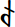-1 Diesis Flat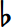-2 Chromatic Semitone Sesquiflat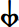or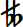-3 Diatonic Semitone Double Flat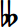-4 Neutral Second

## Understanding Notation: A C-major Scale as Example

Consider how a C-major scale works in 31-ET: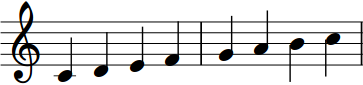This scale consists of whole steps and diatonic semitones. The diatonic semitones are between E and F, and between B and C; all the remaining steps are whole tones.

We avoid using the term "half-step" because in 31-ET, the whole steps cannot be divided equally in half; the whole step must be divided into two distinct semi tones which are unequal in size. In 31-ET, whole steps consist of five steps of the scale, and diatonic semitones consist of 3 steps. Thus, between two notes a whole tone apart, such as D and E, there are 4 intermediate notes, and between two notes a diatonic semitone apart, there are 2 intermediate notes. These notes can be notated using semisharps and semiflats.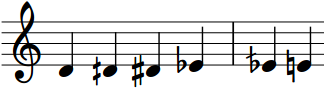Viewed in a table, these notes are as follows:

 D E F D? D# Eb Ed E? E#

There are many different enharmonic equivalents in 31-ET, which are different from the equivalences in 12-ET. The enharmonic equivalents familiar from 12-ET, like E-flat and F-sharp, are not equivalent in 31-ET.